Google Sheets COUNTIF is one of the easiest functions to learn and one of the handiest to use.

It's time to pick up some knowledge on how COUNTIF is used in Google Spreadsheet and learn why this function makes a true Google Spreadsheet companion.

## What is the COUNTIF function in Google Sheets?

This short helper allows us to count how many times a certain value appears within a specified data range.

## COUNTIF syntax in Google Sheets

The syntax of our function and its arguments are as follows:

=COUNTIF(range, criterion)
• range — a range of cells where we want to count a certain value. Required.
• criterion or searching criterion — a value to find and count across the data range indicated in the first argument. Required.

It may seem that COUNTIF is so simple that it doesn't even count as a function (pun intended), but in truth its potential is quite impressive. Its searching criteria alone is enough to earn such a description.

The thing is that we can decide to look for not only concrete values but also those that meet certain criteria.

It's high time to try and build a formula together.

Let's suppose your company sells various types of chocolate in several consumer regions and works with many clients.

This is how your sales data look like in Google Sheets: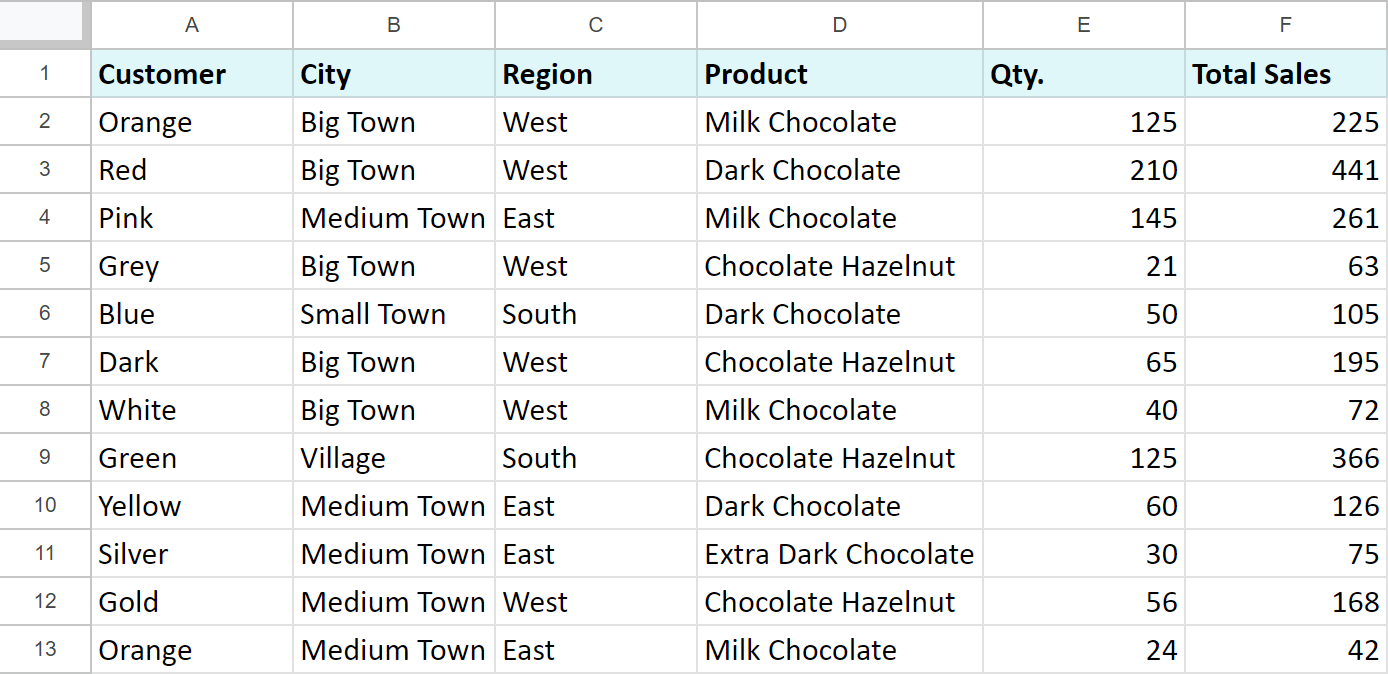Let's begin with the basics.

We need to count the number of "Milk Chocolate" sold. Place the cursor in the cell where you want to get the result and enter the equality sign (=). Google Sheets immediately understands that we are going to enter a formula. As soon as you type the letter "C", it will prompt you to choose a function that begins with this letter. Select "COUNTIF".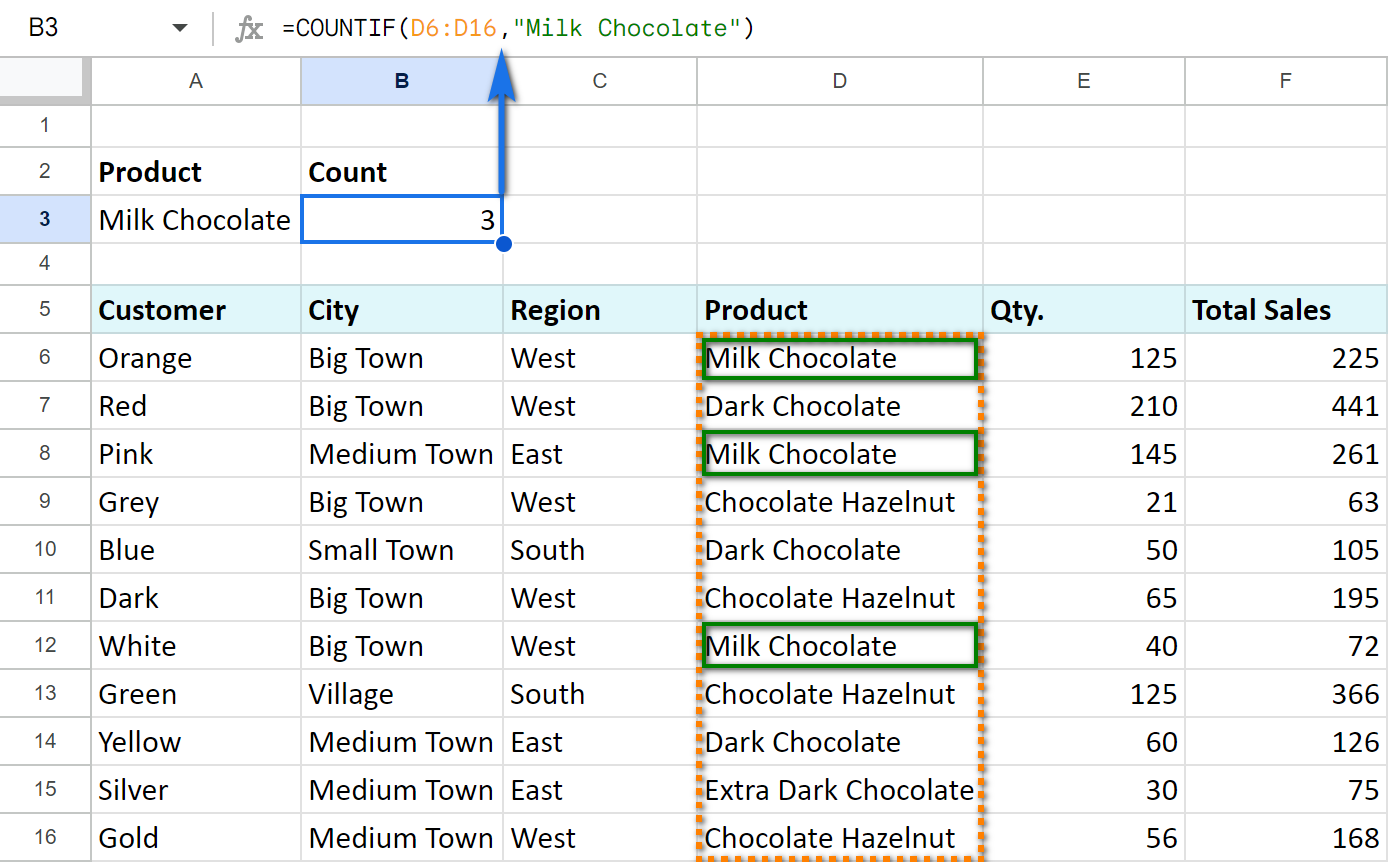The first argument of COUNTIF is represented by the following range: D6:D16. By the way, you don't have to enter the range manually — mouse selection is enough. Then enter a comma (,) and specify the second argument — searching criteria.

The second argument is a value that we're going to look for across the selected range. In our case it's going to be the text — "Milk Chocolate". Remember to finish the function with a closing bracket ")" and press "Enter".

Also, don't forget to enter double quotes ("") when using text values.

Our final formula looks as follows:

`=COUNTIF(D6:D16,"Milk Chocolate")`

As a result, we get three sales of this type of chocolate.

Tip. To count words and characters in Google Sheets, visit this tutorial.

Note. COUNTIF function works with a single cell or neighboring columns. In other words, you can't indicate a few separate cells or columns and rows. Please see the examples below.

Incorrect formulas:

`=COUNTIF(C6:C16, D6:D16,"Milk Chocolate")`

`=COUNTIF(D6, D8, D10, D12, D14,"Milk Chocolate")`

Correct usage:

`=COUNTIF(C6:D16,"Milk Chocolate")`

`=COUNTIF(D6,"Milk Chocolate") + COUNTIF(D8,"Milk Chocolate") + COUNTIF(D10,"Milk Chocolate") + COUNTIF(D12,"Milk Chocolate") + COUNTIF(D14,"Milk Chocolate")`

You may have noticed that it's not really convenient to set the searching criteria in the formula — you have to edit it every time. The better decision would be to write the criteria down other Google Sheets cell and reference that cell in the formula.

Let's count the number of occurred sales in the "West" region using the cell reference in COUNTIF. We'll get the following formula:

`=COUNTIF(C6:C16,A3)`

The function uses the content of A3 (the text value "West") in its calculations. As you can see, it's a lot easier now to edit the formula and its searching criteria.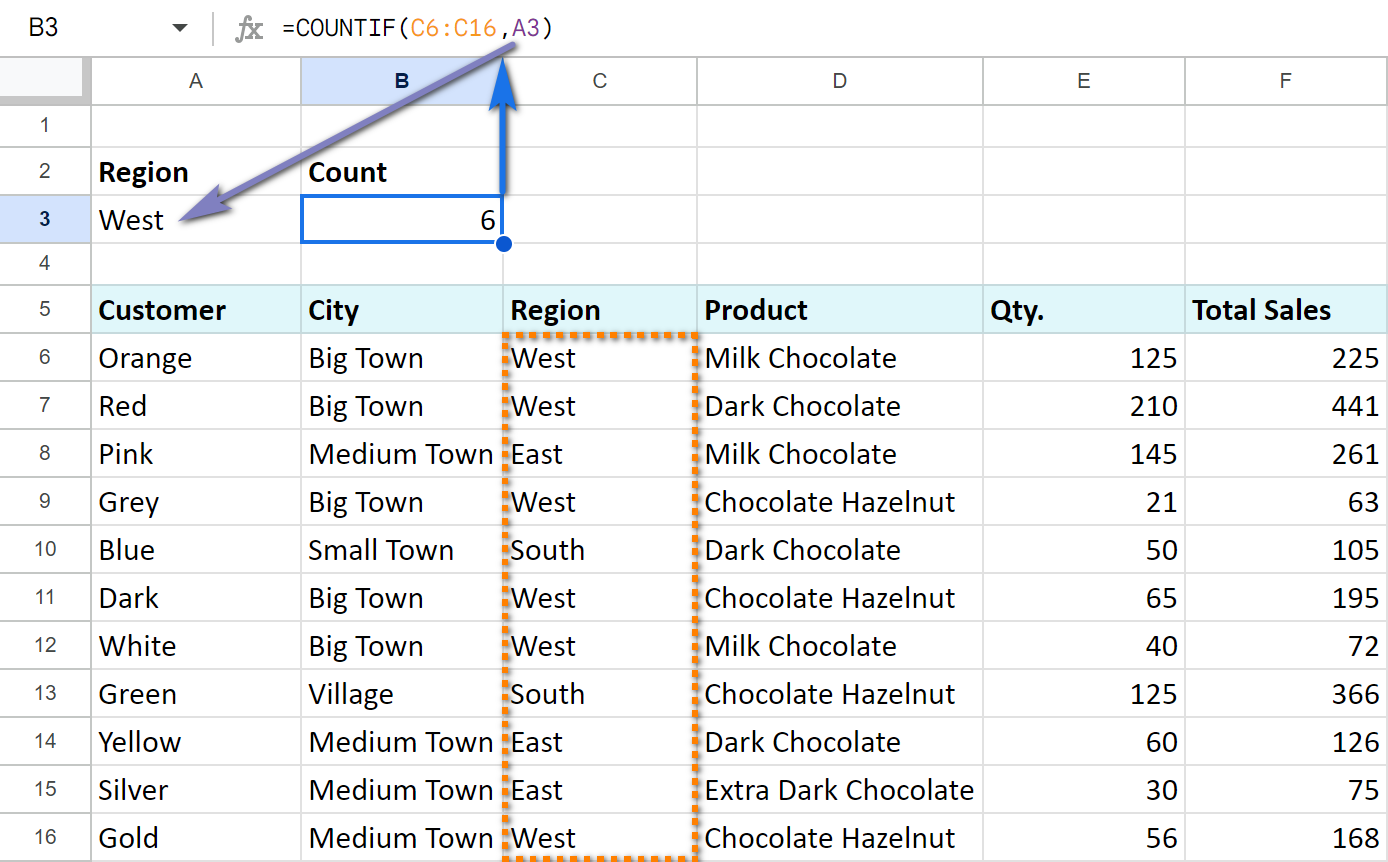Of course, we can do the same thing with numerical values. We can count the number of occurrences of the number "125" by indicating the number itself as a second argument:

`=COUNTIF(E6:E16,125)`

or by replacing it with a cell reference:

`=COUNTIF(E6:E16,A3)`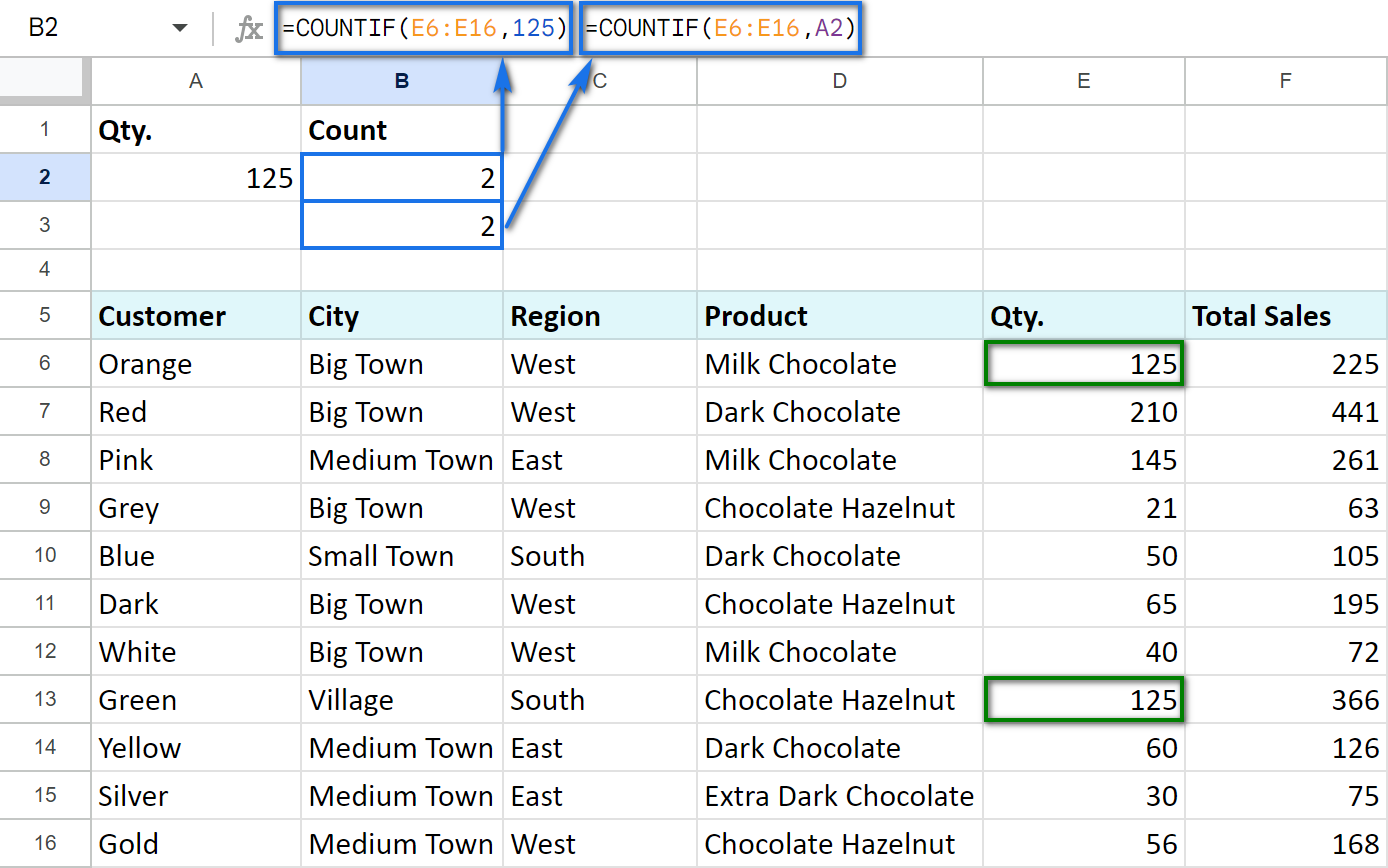What is great about COUNTIF is that it can count whole cells as well as parts of the cell's contents. For that purpose, we use wildcard characters: "?", "*".

For instance, to count the sales in some particular region we can use only part of its name: enter "?est" into A2. A question mark (?) replaces one character. We are going to look for the 4-letter words ending with "est", including spaces.

Use the following COUNTIF formula in B2:

`=COUNTIF(C6:C16,A2)`

As you already know, the formula can easily take the next form:

`=COUNTIF(C6:C16, "?est")`

And we can see 6 sales in the "West" region.

Now let us employ the B3 cell for another formula:

`=COUNTIF(C6:C16,A3)`

What is more, we'll change the criteria to "??st" in A3. It means that now we are going to look for 4-letter words ending with "st". Since in this case two regions ("West" and "East") satisfy our criteria, we will see 9 sales: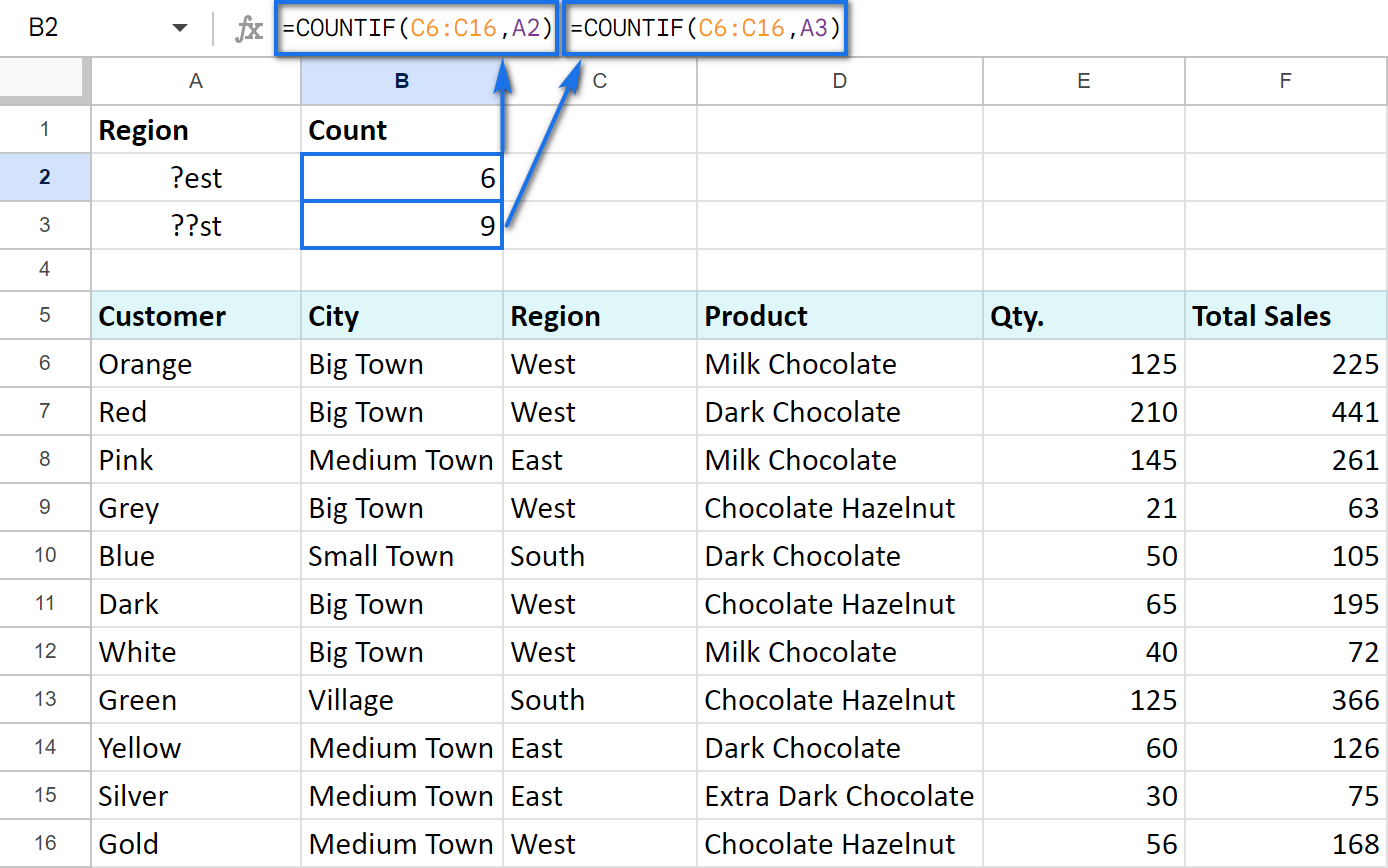Similarly, we can count the number of sales of the goods using an asterisk (*). This symbol replaces not just one, but any number of characters: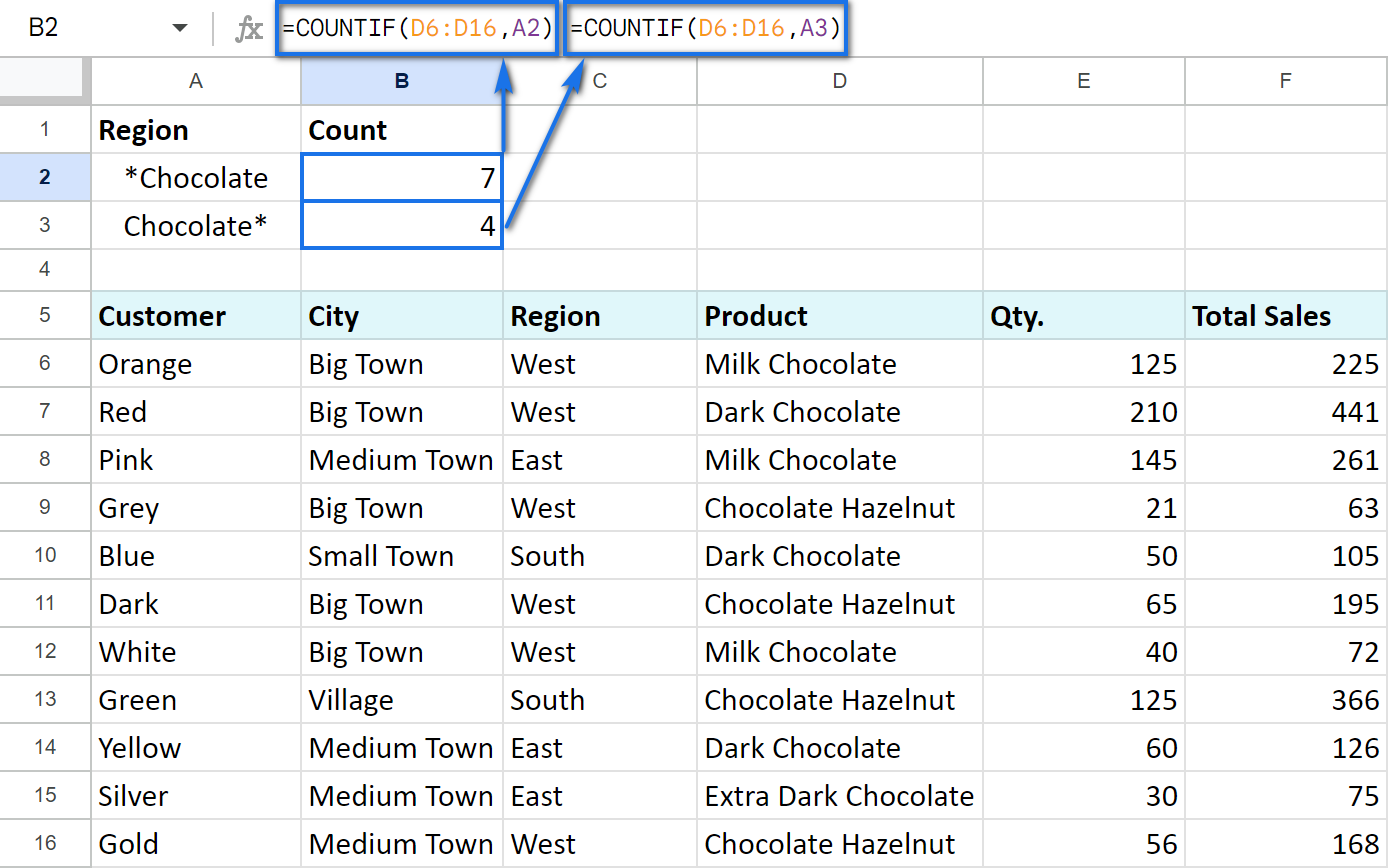`=COUNTIF(D6:D16,A2)`

"*Chocolate" in A2 to count all the products ending with "Chocolate".
`=COUNTIF(D6:D16,A3)`
"Chocolate*" in A3 to count all the products starting with "Chocolate".

And, as you may have guessed, if you enter "*Chocolate*", you're going to look for all the products that contain the word "Chocolate".

Note. If you need to count the number of words that contain an asterisk (*) and a question mark (?), then use tilde sign (~) before those characters. In this case, COUNTIF will treat them as simple signs rather than searching characters. For example, to look for the values that contain "?", the formula will be:

`=COUNTIF(D6:D16,"*~?*")`

### COUNTIF Google Sheets for less than, greater than or equal to

The COUNTIF function is able to count not only how many times some number appears, but also how many of the numbers are greater than/less than/equal to/not equal to another specified number.

For that purpose, we use corresponding mathematical operators: "=", ">", "<", ">=", "<=", "<>".

Check out the table below to see how it works:

 Criteria Formula example Description The number is greater than `=COUNTIF(F7:F16,">100")` Count cells where values are greater than 100. The number is less than `=COUNTIF(F7:F16,"<100")` Count cells where values are less than 100. The number equals to `=COUNTIF(F7:F16,"=100")` Count cells where values equal to 100. The number is not equal to `=COUNTIF(F7:F16,"<>100")` Count cells where values are not equal to 100. The number is greater than or equal to `=COUNTIF(F7:F16,">=100")` Count cells where values are greater than or equal to 100. The number is less than or equal to `=COUNTIF(F7:F16,"<=100")` Count cells where values are less than or equal to 100.

Note. It's very important to enclose the mathematical operator along with a number in the double quotes.

If you want to change the criteria without altering the formula, you can reference the cells as well.

Let me reference A2 and put the formula in B2, just as I did before:

`=COUNTIF(F7:F16,A2)`

To create more sophisticated criteria, use an ampersand (&).

For example, B3 contains a formula which counts the number of values greater than or equal to 100 in the E7:E16 range:

`=COUNTIF(E7:E16,">="&A3)`

B4 has the very same criteria, but we reference not only the number in that cell but also a mathematical operator. This makes it even easier to adapt COUNTIF formula if necessary:

`=COUNTIF(E7:E16,A4&A3)`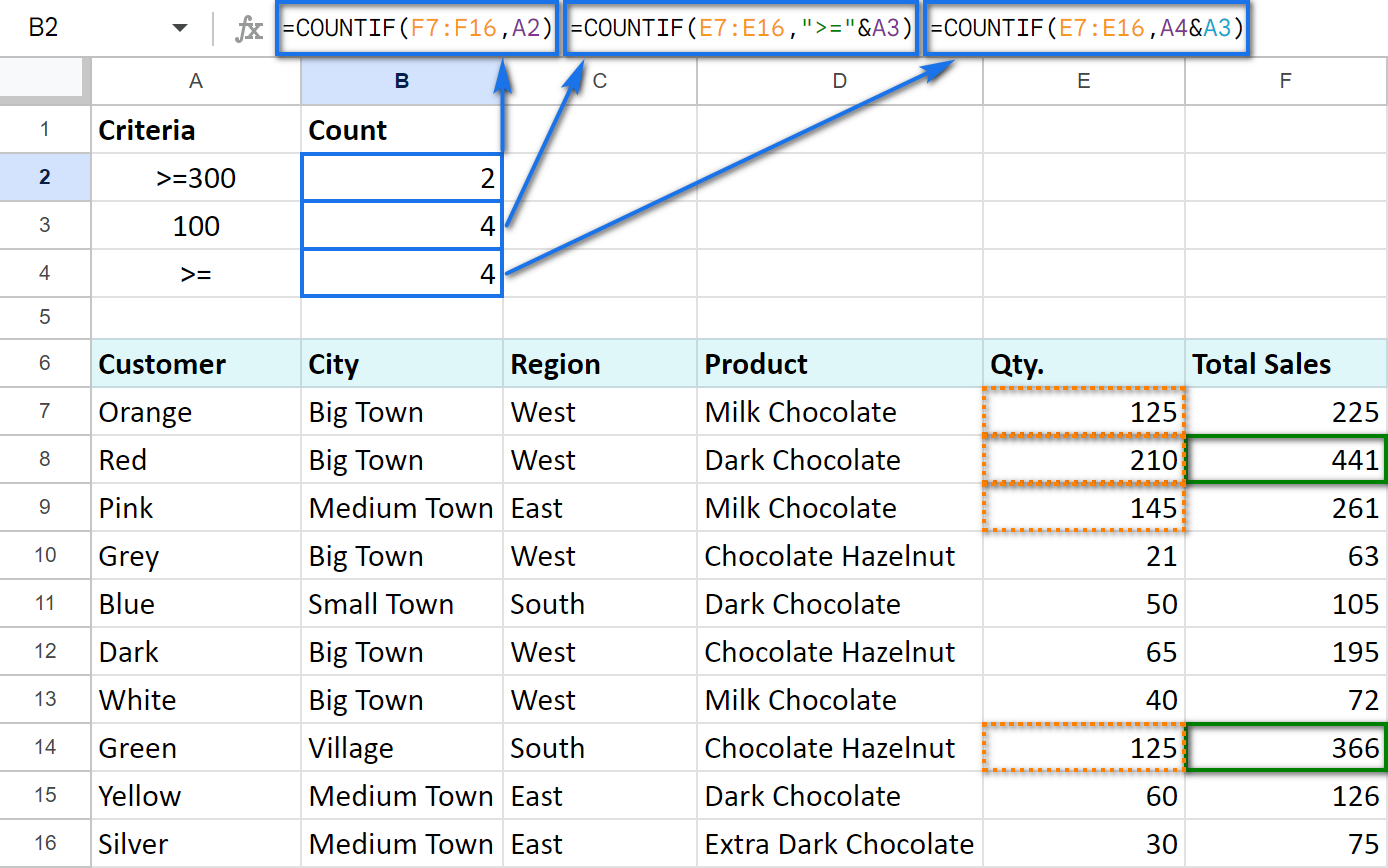Tip. We've been asked a lot about counting those cells that are greater than or less than values in another column. If that's what you're looking for, you will need another function for the job — SUMPRODUCT.

For example, let's count all rows where sales in column E are bigger than in the same row of column F:

`=SUMPRODUCT(--(E6:E17>F6:F17))`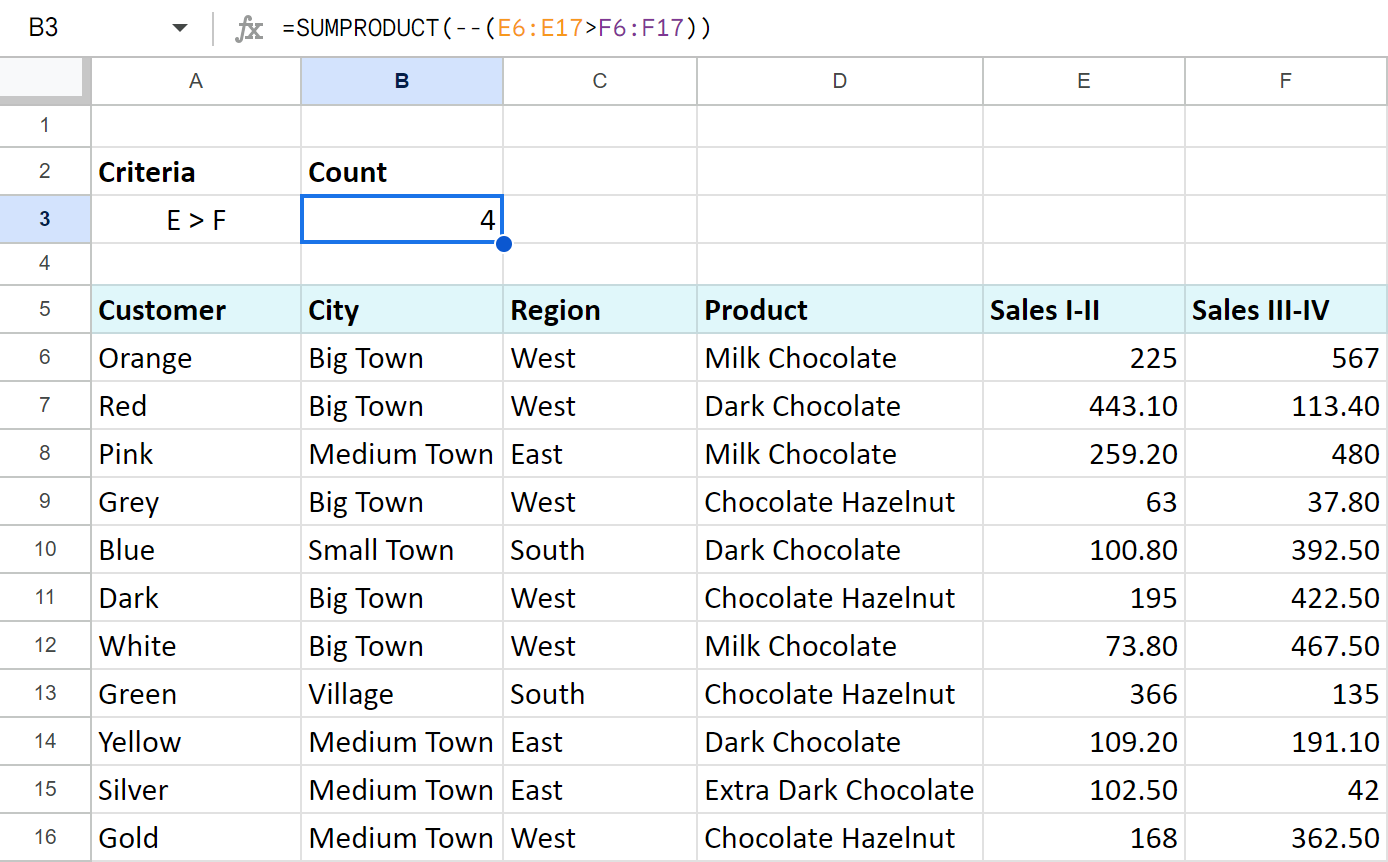• The part at the core of the formula — E6:E17>F6:F17 — compares values in columns E and F. When the number in column E is greater, the formula takes it as TRUE, otherwise — FALSE.

You'll see that if you enter the same into the ArrayFormula:

`=ArrayFormula(E6:E17>F6:F17)`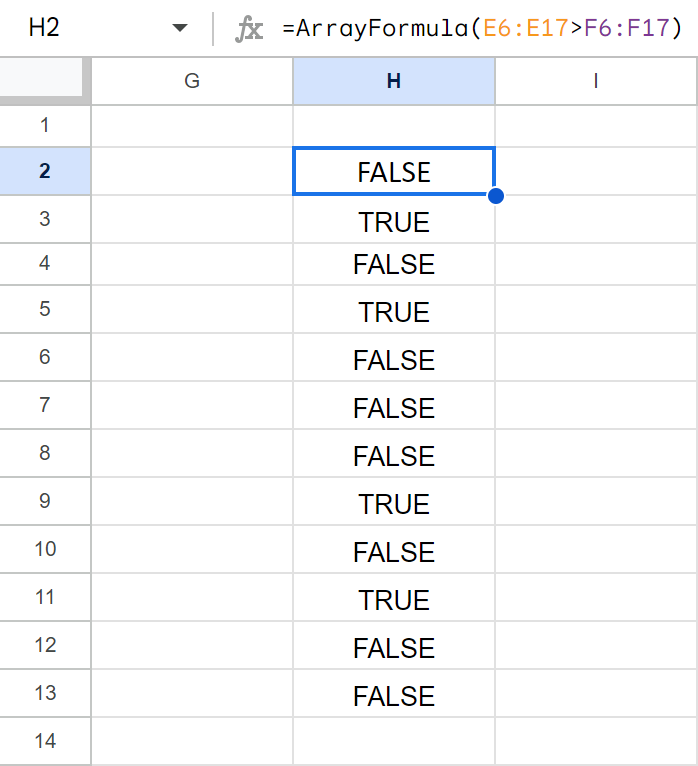• Then the formula takes this TRUE/FALSE result and turns it into 1/0 numbers with the help of the double unary operator (--).
• This lets SUM do the rest — total the number of when E is greater than F.

Sometimes it's necessary to count the number of values that answer at least one of the mentioned conditions (OR logic) or multiple criteria at once (AND logic). Based on that, you can use either a few COUNTIF functions in a single cell at a time or the alternate COUNTIFS function.

### Count in Google Sheets with multiple criteria — AND logic

The only way I’d advise you to use here is with a special function that is designed to count by multiple criteria — COUNTIFS:

=COUNTIFS(criteria_range1, criterion1, [criteria_range2, criterion2, ...])

It is normally used when there are values in two ranges that should meet some criteria or whenever you need to get the number falling between a specific range of numbers.

Let’s try and count the number of total sales between 200 and 400:

`=COUNTIFS(F5:F16,">=200",F5:F16,"<=400")`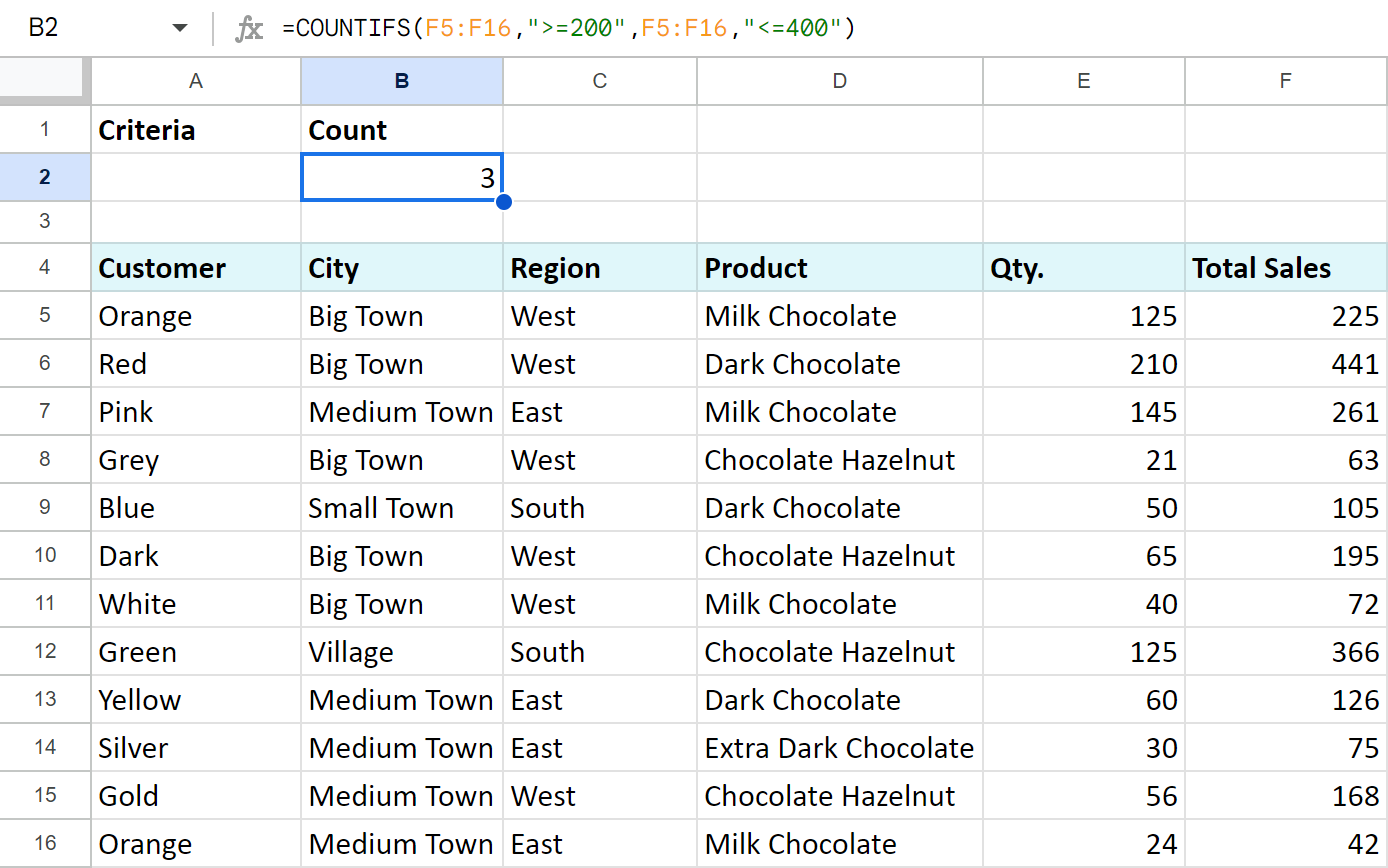### Count uniques in Google Sheets with multiple criteria

You can go further and count the number of unique products between 200 and 400.

Nope, it's not the same as above! :) The above COUNTIFS counts each occurrence of sales between 200 and 400. What I suggest is to also look at the product. If its name occurs more than once, it won't be included in the result.

There's a special function for that — COUNTUNIQUEIFS:

COUNTUNIQUEIFS(count_unique_range, criteria_range1, criterion1, [criteria_range2, criterion2, ...])

Compared to COUNTIFS, it's the first argument that makes the difference. Count_unique_range is that range where the function will count unique records.

Here's how the formula and its result will look:

`=COUNTUNIQUEIFS(D5:D16,F5:F16,">=200",F5:F16,"<=400")`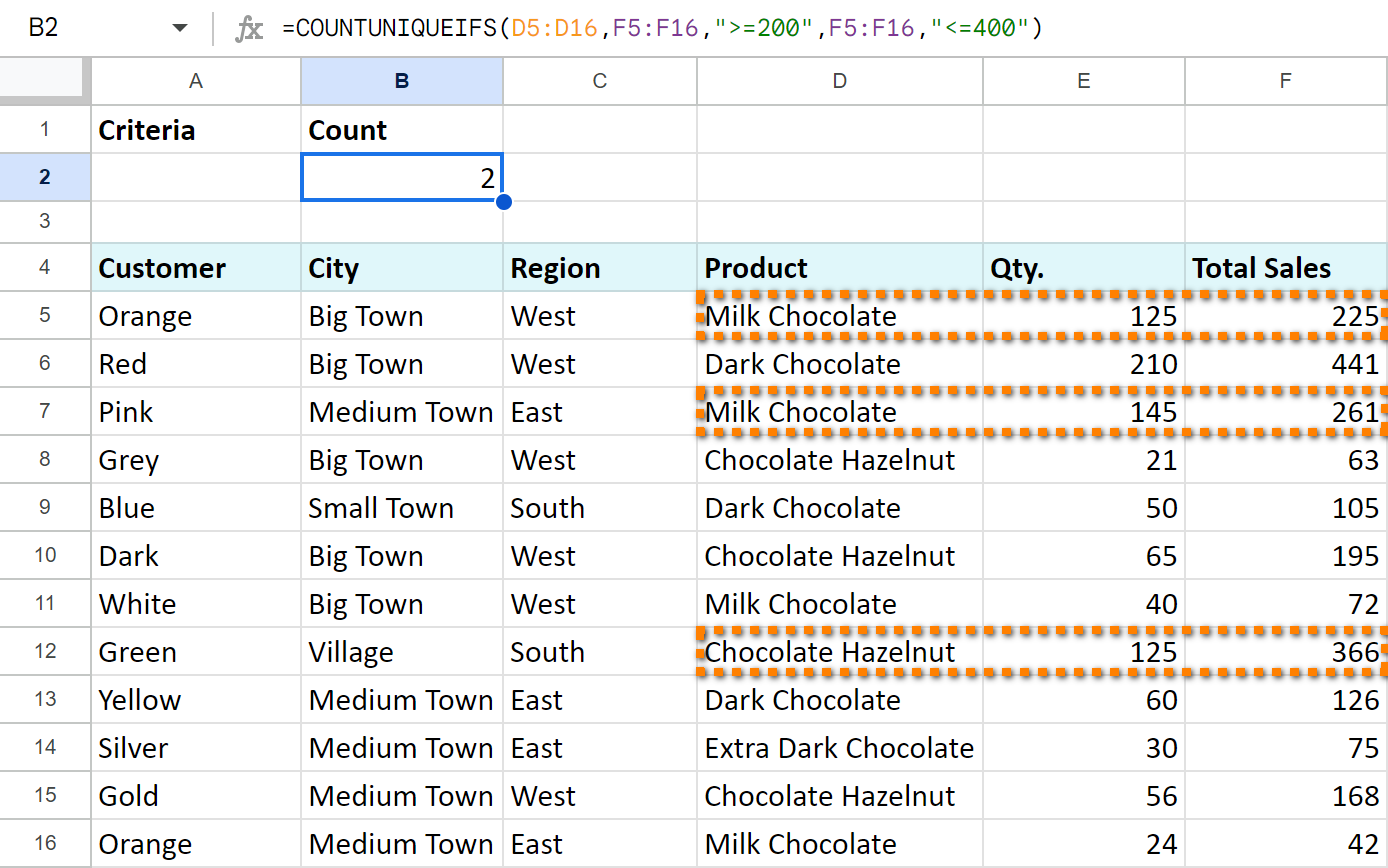Look, there are 3 rows that meet my criteria: the sales are 200 and greater and at the same time are 400 or less.

However, 2 of them belong to the same product — Milk Chocolate. COUNTUNIQUEIFS counts the first mention of the product only.

Thus, I know that there are only 2 products that meet my criteria.

### Count in Google Sheets with multiple criteria — OR logic

When only one of all criteria is enough, you’d better use several COUNTIF functions.

#### Example 1. COUNTIF + COUNTIF

Let's count the number of sales of black and white chocolate. To do that, enter the following formula in B2:

`=COUNTIF(D6:D16,"*Milk*") + COUNTIF(D6:D16,"*Dark*")`

Tip. I use asterisk (*) to ensure that the words "dark" and "milk" will be counted no matter where they are in the cell — at the beginning, in the middle, or at the end.

Tip. You can always introduce cell references to your formulas. See how it looks on the screenshot below in B3, the result remains the same:
`=COUNTIF(D6:D16,A2) + COUNTIF(D6:D16,A3)`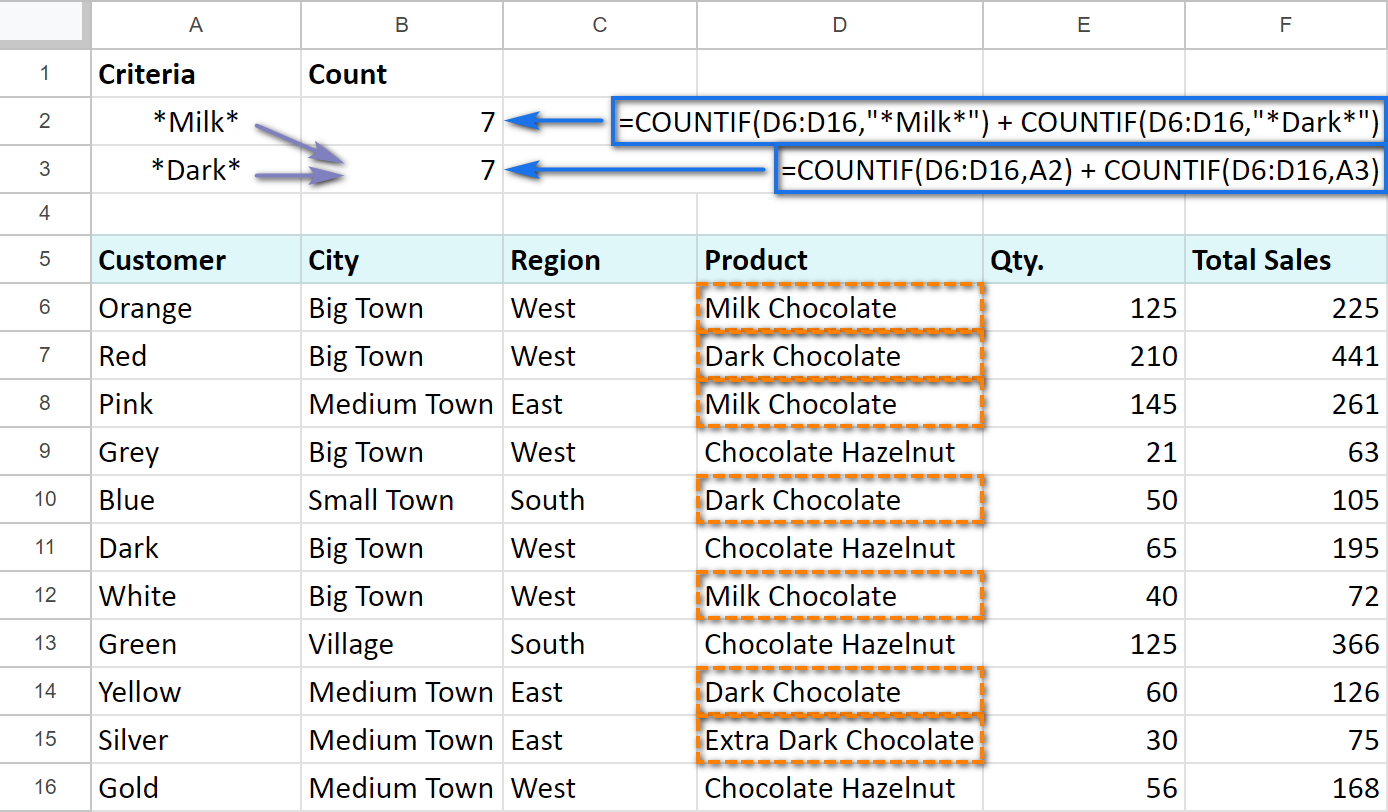#### Example 2. COUNTIF — COUNTIF

Now, I am going to count the number of total sales between 200 and 400: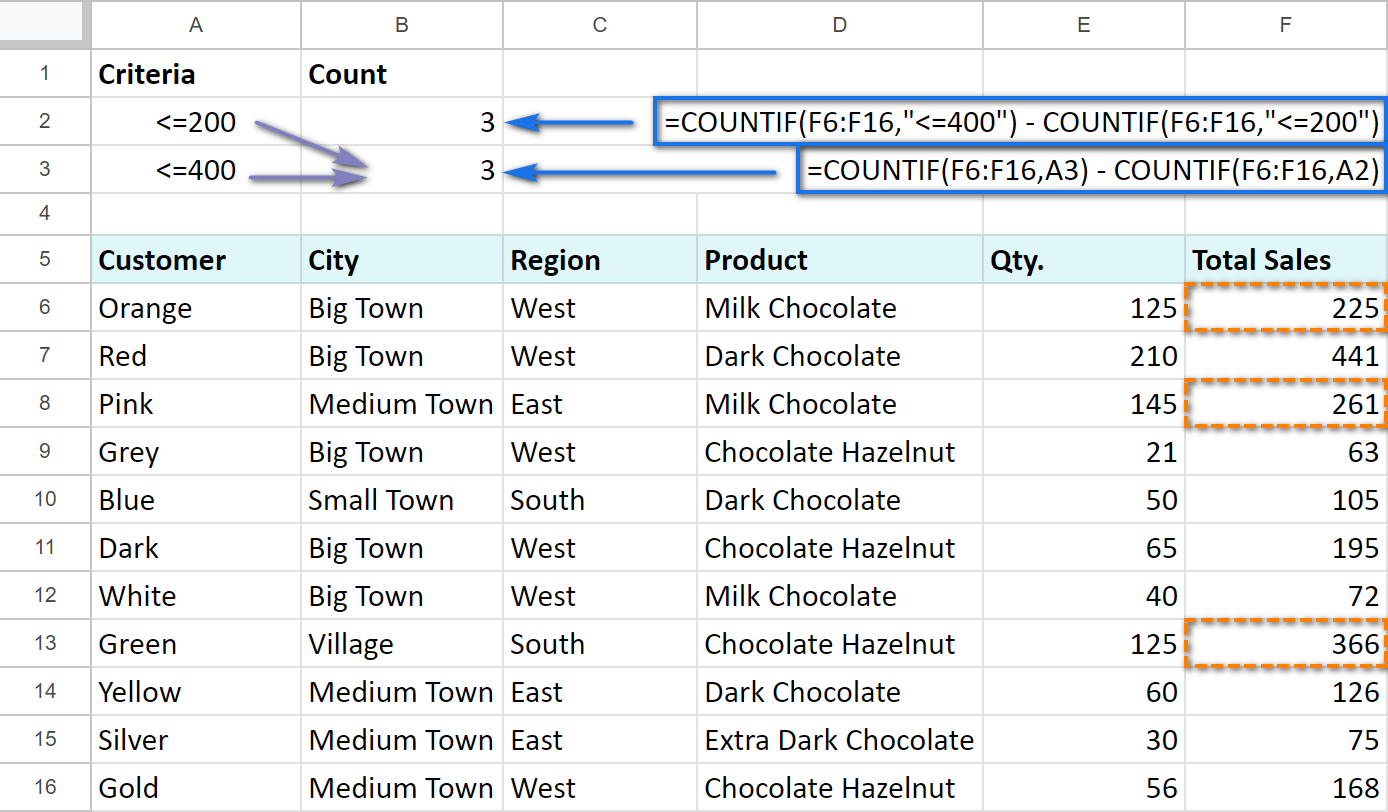I take the number of totals under 400 and subtract the number of total sales under 200 using the next formula:

`=C0UNTIF(F6:F16,"<=400") — COUNTIF(F6:F16,"<=200")`

The formula returns the number of sales more than 200 but less than 400.

If you decide to reference A2 and A3 that contain the criteria, the formula will be a bit simpler:

`=COUNTIF(F6:F16, A3) — COUNTIF(F6:F16, A2)`

A3 cell will have "<=200" criteria, while A4 — "<=400". Put formulas into B2 and B3 and make sure that the result doesn't change — 3 sales over the needed range.

## COUNTIF Google Sheets for blank and non-blank cells

With the help of COUNTIF, we can also count the number of blank or non-blank cells within some range.

Let's suppose that we successfully sold the product and marked it as "Paid". If the customer declined the goods, we write zero (0) in the cell. If the deal wasn't closed, the cell remains empty.

To count non-blank cells with any value, use the following:

`=COUNTIF(F6:F16,"<>")`

or

`=COUNTIF(F6:F16,A2)`

To count the number of empty cells, make sure to put the COUNTIF formula in the following way:

`=COUNTIF(F6:F16,"")`

or

`=COUNTIF(F6:F16,A3)`

The number of cells with a textual value is counted like this:

`=COUNTIF(F6:F16,"*")`

or

`=COUNTIF(F6:F16,A4)`

Screenshot below shows that A2, A3, and A4 cells include our criteria: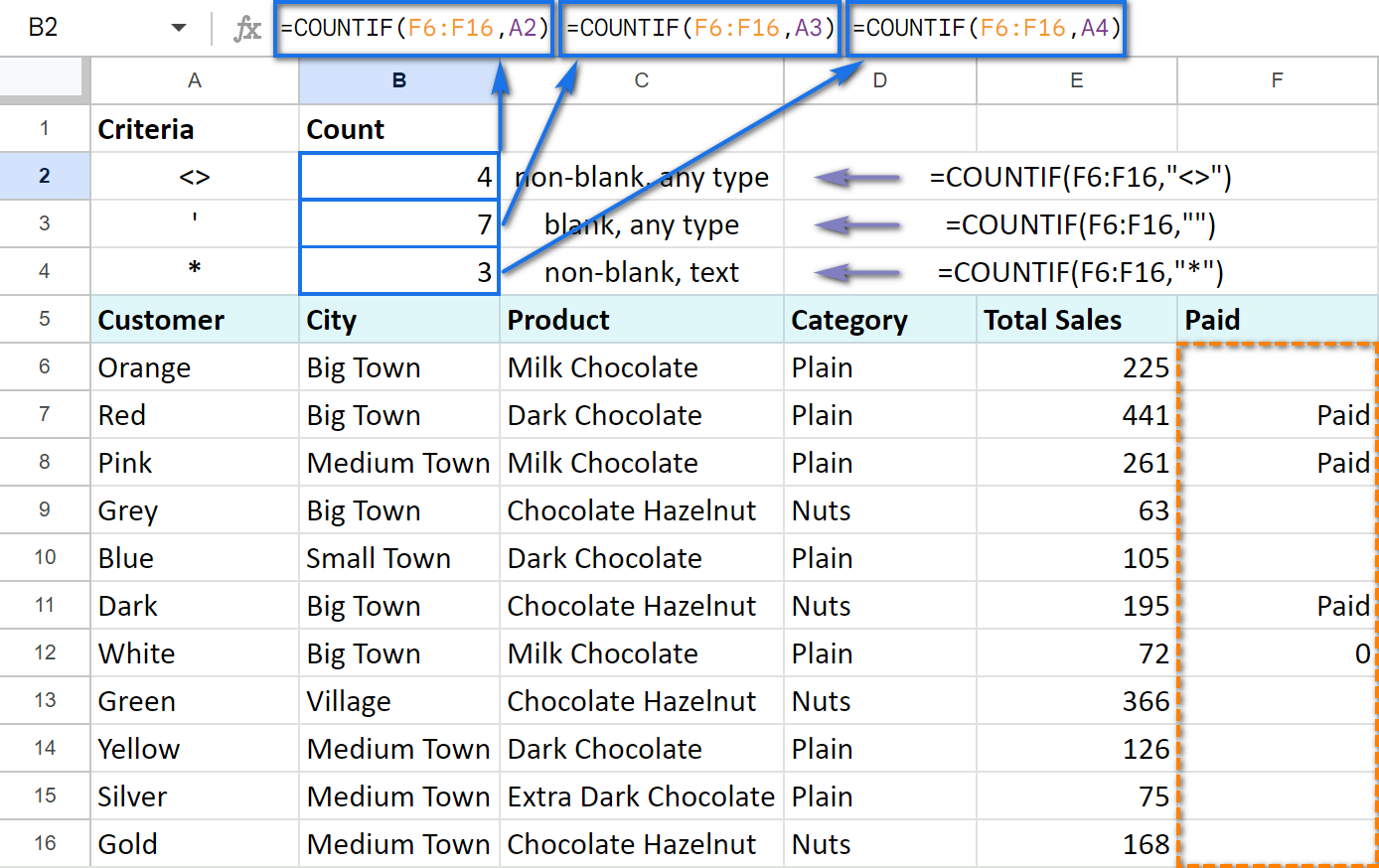Thus, I have 4 closed deals, 3 of which were paid for and 7 of which have no markings yet and, consequently, are not closed.

## COUNTIF and conditional formatting

There is one interesting opportunity that Google Sheets offer — to change the cell's format (like its color) depending on some criteria. For example, we can highlight the values that appear more often in green.

COUNTIF function can play a small part here as well.

Select the range of the cells that you want to format in some special way. Click Format -> Conditional formatting...

In the Format cells if... drop-down list choose the last option Custom formula is, and enter the following formula into the appeared field:

`=COUNTIF(\$B\$8:\$B\$38,B8)/COUNTIF(\$B\$8:\$B\$38,"*")>0.3`

It means that the condition will be answered if the value from B8 appears within B8:B38 in more than 30% of cases: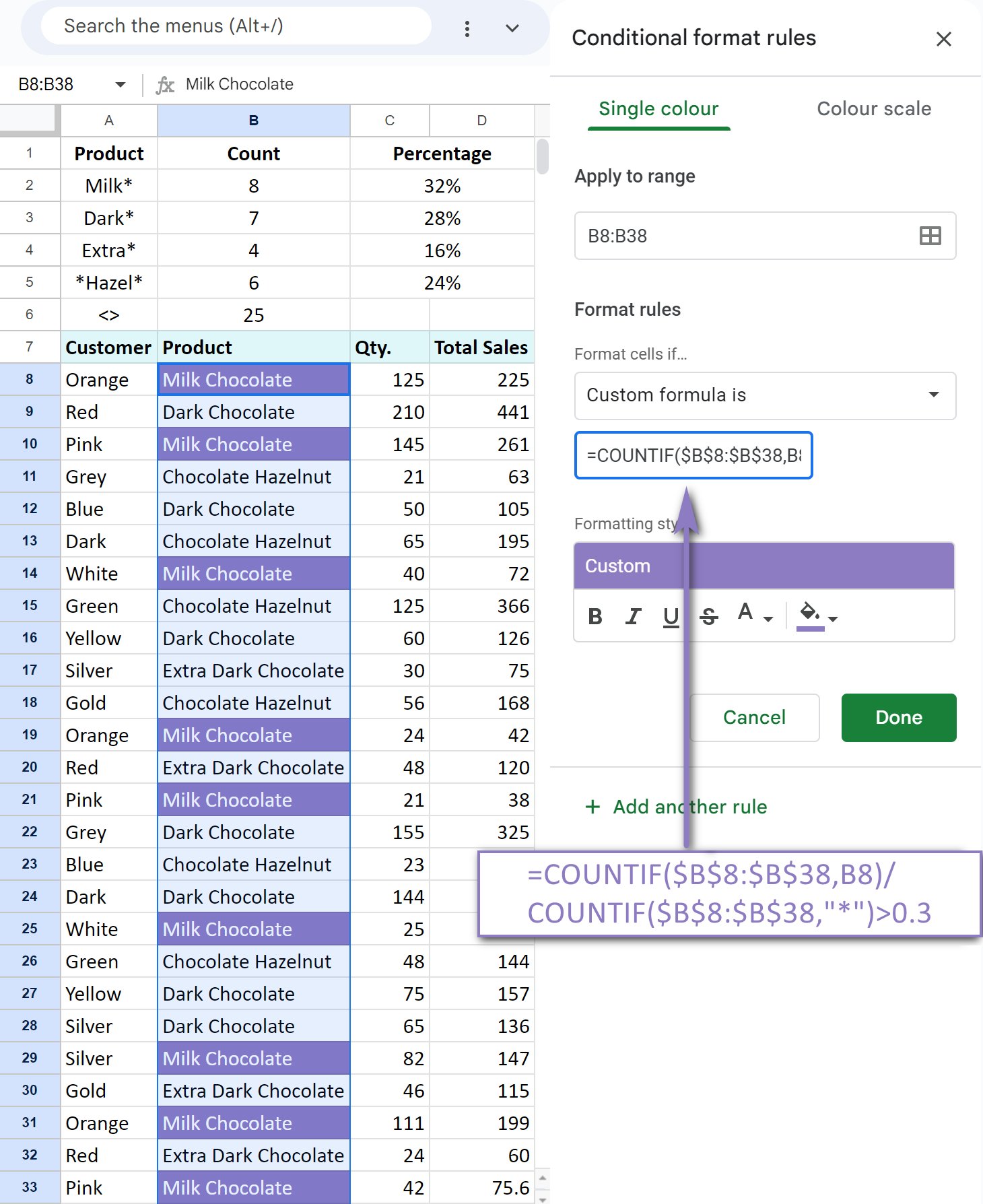In a similar way, we add two more formatting rule criteria — if the cell value appears more often than in 25% of cases and more often than in 20%:

`=COUNTIF(\$B\$8:\$B\$38,B8)/COUNTIF(\$B\$8:\$B\$38,"*")>0.25`

`=COUNTIF(\$B\$8:\$B\$38,B8)/COUNTIF(\$B\$8:\$B\$38,"*")>0.2`

Keep in mind that the first criterion will be checked beforehand, and if it's met, the rest won't apply. That is why you'd better start with the most unique values moving to the most common ones. If the cell value doesn't meet any criteria, its format will remain intact.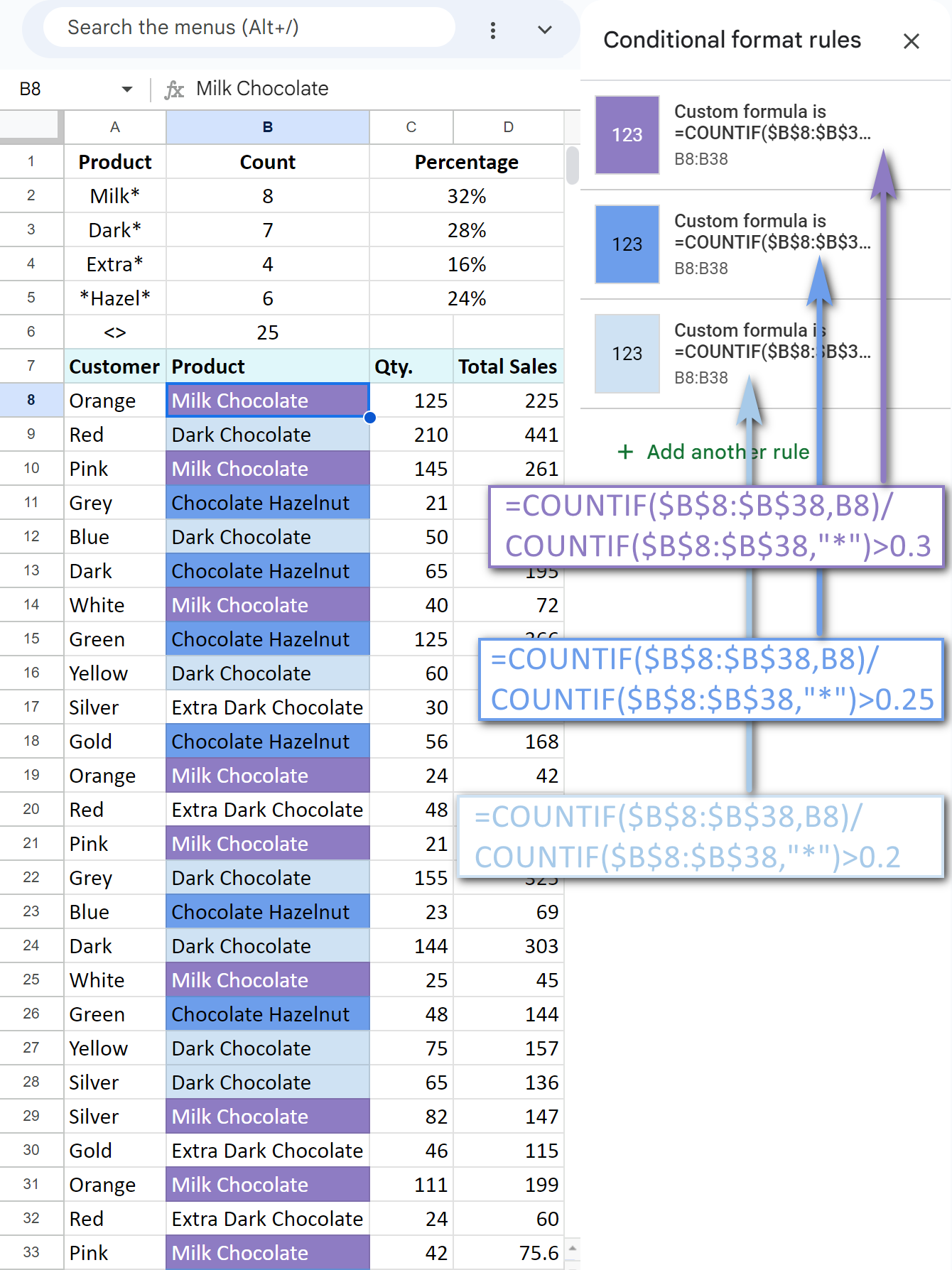You can see that the colour of the cells has changed according to our criteria.

To make sure, we also counted the frequency of some values in C2:C5 using the COUNTIF function. The results confirm that COUNTIF in formatting rule was applied correctly.

Tip. Find more examples on how to count & highlight duplicates in Google Sheets.

All these function examples give us a clear understanding of how Google Spreadsheet COUNTIF offers multiple opportunities to work with the data in a most efficient way.

## COUNTIF color in Google Sheets

One task that gains popularity is counting cells by their color. Though there's no native Google Sheets function to do that, you can still achieve the result without coding. All you need is an add-on from the Google Workspace Marketplace: Function by Color.

• Count cells of any particular font or fill color in your Google Sheet: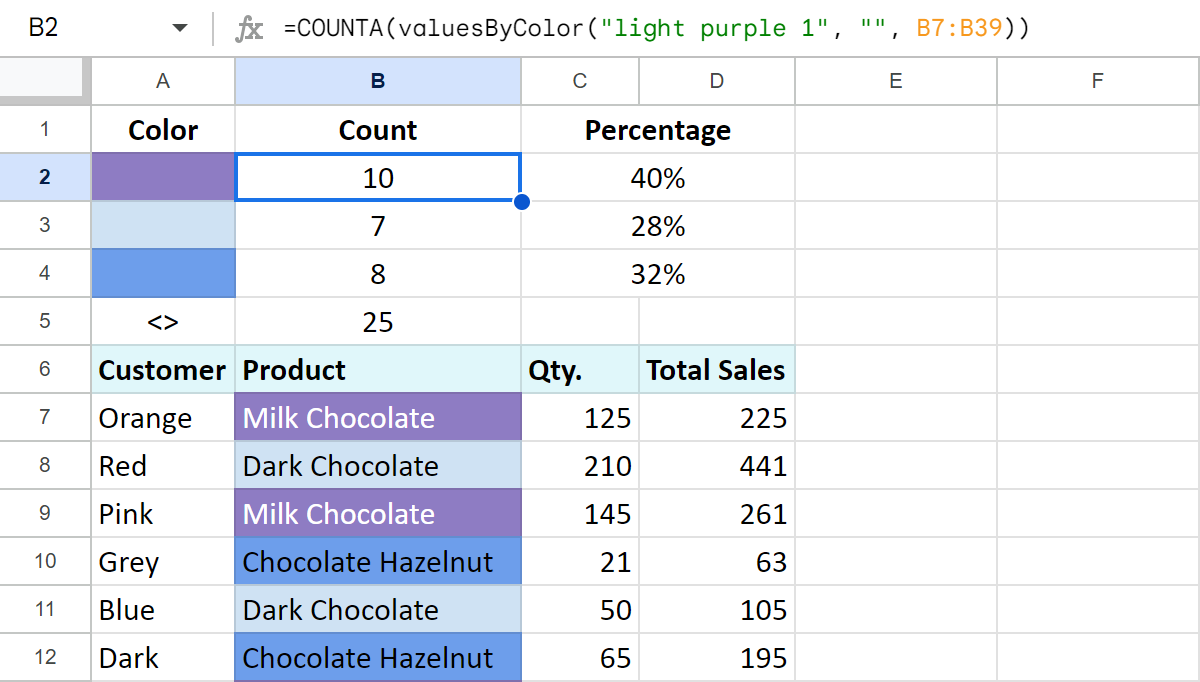• Count all colored cells in the range: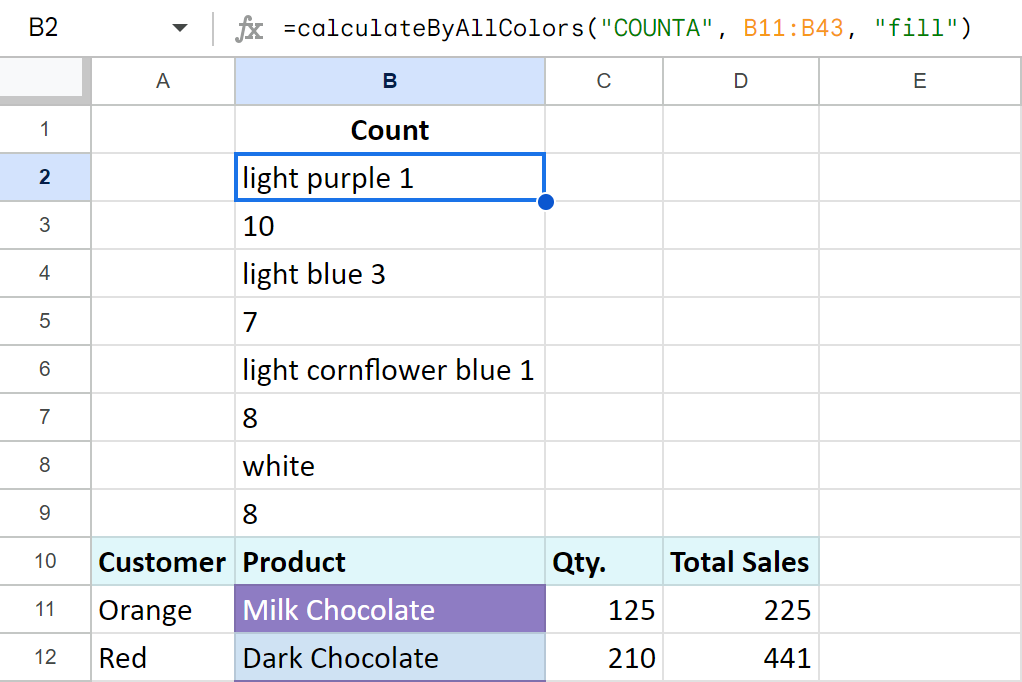• Another ability you gain with Function by Color is using colors as extra conditions in your own COUNTIFS (and other) formulas: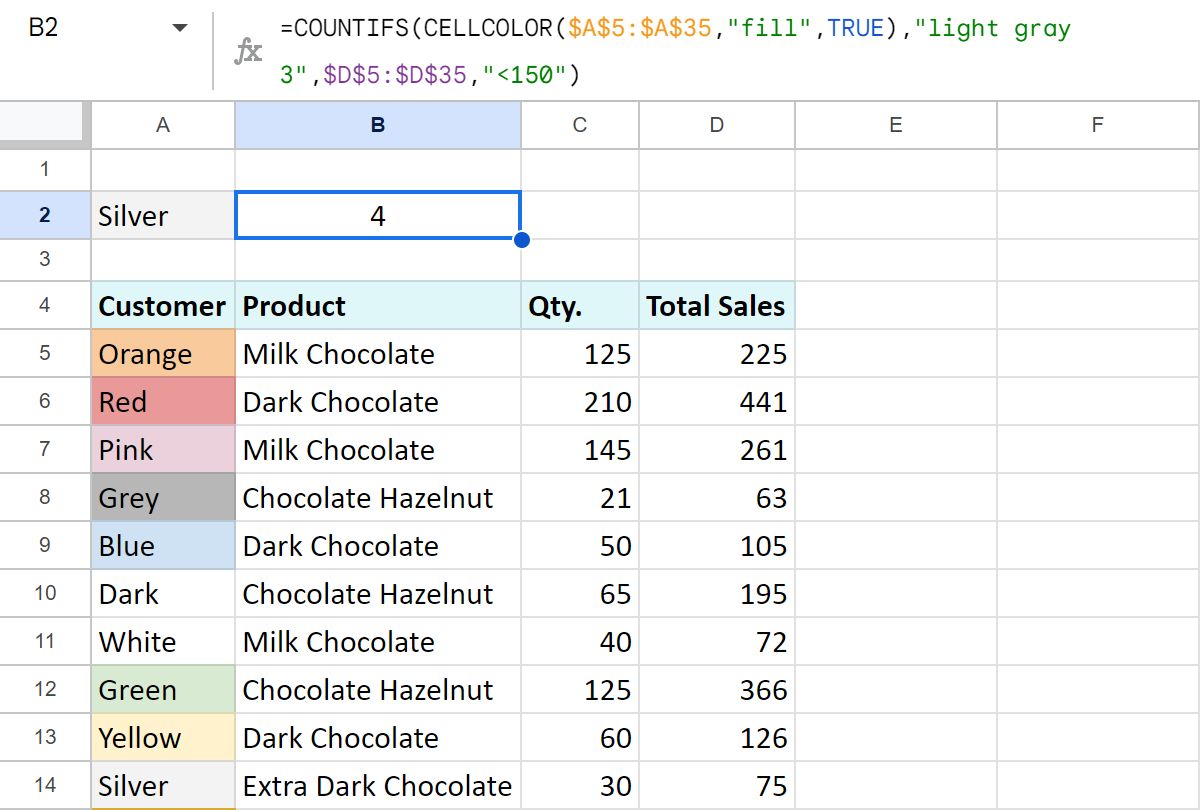The following blog article breaks down this usage to pieces: Functions for Google Sheets to work with colored cells

### Video: How to count colored cells in Google Sheets

Install Function by Color from Google Workspace Marketplace to follow the examples along or to try it on your own cases. Let me know how it goes in the comments section below! :)

## You may also be interested in

1. Hi there!

I'm so grateful for you for writing these articles! I'm stuck on 1 thing. I'm trying to create a formula to repeat a fixed value every 3 months for X times.
I think I have the formula to repeat the fixed value every 3 months, but I'm not sure on how to "end" the calculation with how many times it needs to run. Ie. I receive a payment of \$200 every 3 months, which will happen 5 times.

Here's the formula I'm working with right now; =IF(F18=start,(MOD(DATEDIF(start,F18,"m")+D19,D19)=0)*200,0)

F18 is the date on the calendar (going by months)
Start is the day payments started
D19 is the cell that indicates every 3 months
And 200 is the value

But how do I close this string off after so many times?

Thank you! Have an amazing day!

• Hi! If I understand your task correctly, to create a sequence of dates with an interval of 3 months from the start date, try this formula:

=ARRAYFORMULA( date(YEAR(\$F\$18),MONTH(\$F\$18)+SEQUENCE(5,1,0,3),day(\$F\$18)))

2. I have a mixed list of middle and high schoolers and I want to be able to provide an at-a-glance number of how many of each are in my table.

Essentially, I'd like a formula that counts the number of times "9th" "10th" "11th" and "12th" appear in the column while ignoring the "6th" "7th" and "8th" values, and vice versa, so that I can have separate outputs for the number of middle schoolers and the number of high schoolers in my dataset.

Thanks!

• Hello Chris,

COUNTIF described above is perfect to count pupils from each class.
If you'd like to count the total from 4 grades, you'll need to sum a few COUNTIF formulas, like in the example here.

3. Hi, I'm trying to build a formula on google sheet whereby I get a total number of cases solved by an a person during a specific week (06/29/2023 - 07/02/2023). I've used multiple formulas and am either getting 0 or getting incorrect numcers. I'm using two tabs, and I'm using the following formula:

=COUNTIFS('Copy of Solve Count DATA'!E:E,\$A\$60,'Copy of Solve Count DATA'!D:D,">=2023/06/26",'Copy of Solve Count DATA'!D:D,"<=2023/07/02")

Copy of Solved Count DATA! E:E contains the names of all agents.
\$A\$60 is the tab I need the formula in, and that cell contains the name of one specific agent.
Copy of Solve Count DATA'!D:D contains the all the dates from January till now

Am I using the correct formula or am I way off?

Thank you!

• Hello Mari,

Use the DATE function for the dates as conditions. Please see the example in this comment.

4. Hi,
I have formulas in cells:
O5
H7+(H7*25%)

And P5
=H7-(H7*25%)

Trying to determine whether the value in cell D7 falls between the above range. I've tried

IF(AND(\$D7>\$O5,\$D7\$O5,\$D7<\$P5,"Yes","No")- Wrong number of argument
Thanks

• Hi Mahdee,

You forgot to close the AND argument right before "Yes".
There's also some comparison operator missing in \$D7\$O5.

5. Hi,
I have a Problem! i want to count Cells, where the Data is "> 50.000 €". But when i'm using criterion > 50.000 € it counts the "bigger" ones, so it is 0! I have tested many options, bit don't get the right Data...

• For better explanation:

I have a Sheet with 5 different Values in B:B: 50.001 €.

If I use:
=COUNTIF(B:B;"15.001 € - 30.000 €")

everything works fine. But if I use:
=COUNTIF(B:B;" 50.001 €")

i already have tried to use
=COUNTIF(B:B ; B3)

because I know, that B3 is the Value i'm looking for. But the answer is still "0"!

• Hi Max,

Please consider sharing an editable copy of your spreadsheet with us (support@apps4gs.com) with the result you expect to get and the formulas you tried.
Note. We keep that Google account for file sharing only and don't monitor its Inbox. Please do not email there. Once you share the file, just confirm by replying to this comment.

6. Hi ,
I am a google sheet user. i want to automate the sum function in my sheet. there are so many data. it is really difficult to calculate manually .

A B C D
Chair 69,626 55,892 138,376 106,865
Table 16,089 13,336 31,400 26,105
Accessories 4,321 3,369 8,537 6,737
Total

Art 200,692 30,667 170,968 2,530
Mirrors 278,902 -21,260 130,890 2,594/
Fragrances 102,283 4,304 47,232 2,678
Lamps 11,735 1,132 25,469 1212
Floral 23456. 2345. 30000 23125
Total

• Hi Twincky,

For me to be able to help you, please specify what total should return and why the SUM function doesn't work for you.

7. Can Countif be used in an array formula to count along rows how many times a cell value occurs in that row so that there is no need to drag the formula down each cell in a column

Example formula that gives single result countif(B2:G2, "Y")

• Hello Roger,

=ARRAYFORMULA(SUM(IF(\$B11:\$D11="Y",1,0)))

8. I am using Google Sheets and using Query to pull one column of data from three tabs. The data is text and is not in any order.

1. I am trying to look for exact match text duplicates in the columns - same column or any of the three
- =COUNTIF(\$B\$2:\$D\$1000,B2)>1 seems to identify partial matches, like certain letter combinations or words

2. The Query works great and brings in new additions to the columns when they are added in real time but if I enter two cells of the same text, the Countif does not seem to catch the matched text even if I refresh the browser

Input welcome, thanks!

Kevin

• Hello Kevin,

Please consider sharing an editable copy of your file with us directly: support@apps4gs.com
Please include the formulas that you tried to use. If you have confidential information there, you can replace it with some irrelevant data, just keep the format.

Note. We keep that Google account for file sharing only and don't monitor its Inbox. Please do not email there. Once you share the file, just confirm by replying to this comment.

I'll look into it and try to help.

9. Hi there,

I've asked for help before with developing a formula that counts the number of times "Cat" is input based off of the date selected in the past on a different page and the formula given to me that worked was:

=SUMPRODUCT(--('2023-2024'!A:A=DATE(2023, 5,10)),--(ISNUMBER(SEARCH("Cat",'2023-2024'!H:H))))

Other sheet name: 2023-2024
Other sheet columns to count: H:H "Cat"
Other sheet columns to add to count: G:G "Black"

I'm now looking for the same formula but looking to add another criteria to it so I'm looking to count (from a different sheet) that on a certain date, if the criteria in H:H has the text "Cat" and G:G has the text "Black" what the answer would be.

Date > H:H Column > G:G Column = Count

• Hi Cory,

I can see your previous question was for Excel. Do you work in Excel or Google Sheets? It's important since functions differ based on the platform.

• Hi Natalia,

Thanks!

• Hi Cory,

Okay :) Here's the updated formula (with an additional ISNUMBER/SEARCH condition at the end):
=SUMPRODUCT(--('2023-2024'!A:A=DATE(2023, 5,10)),--(ISNUMBER(SEARCH("Cat",'2023-2024'!H:H))),--(ISNUMBER(SEARCH("Black",'2023-2024'!G:G))))

• Thank you!!!

• You're most welcome!

10. Hello!

I'm trying to use conditional formatting using drop-down list cells in column D beginning with cell D2. I want all cells to turn yellow when more than one of the same item is chosen from the drop down lists in that column. I'm using yellow as a warning sign to users when an item from the column has already been chosen and shouldn't be selected again. This conditional formatting formula gets me most of the way there:

=COUNTIF(\$D\$2:\$D,\$D2)>1

The problem I'm having is that the formula above also turns all the cells with blank selections yellow, presumably because the blank cells match each other, so the formula thinks (correctly) that the blanks have been duplicated at least once! What formula allows me to indicate duplicate selections within a column by turning those cells yellow while excluding duplicate blanks?

• Brilliant! That did it!

Can't thank you enough!

• Happy to help! :)

11. Hi,

In my sheet I have in columns:

A - dates (yymmdd)
B - types of things (lets say apples, oranges, pears)

Now if I want to count, in a seperate cell, the number of "oranges" registered during the year 2021, how can I do that?
Seems like it should be easy but I can't make it work.

Help?

• Hi JN,

To count by several criteria at once, use COUNTIFS described in this part of the article.
To set up the whole year, you'll need to build 2 criteria like this:
=COUNTIFS(A:A,">="&DATE(2021,1,1),A:A,"<="&DATE(2021,12,31),B:B,"orange")

• Thank you so much!
It worked. :)

• You're most welcome! Happy to help :)

12. Hi, i am using COUNTIF formula with conditional formatting for Highlight duplicates in data (Number)
=COUNTIF(\$A:\$A,\$A1)>1 ,
Now i need to highlight duplicate by checking the last 4-5 numbers in a cell of my perspective data and highlight it,

• Hi Alex,

Am I getting it right that your numbers should be marked as duplicates only if their 4-5 last digits match?

• Yes, Natalia

• In this case, COUNTIF won't do for the conditional formatting. Here's another formula:
=IF(LEN(G1)>3,IFNA(VLOOKUP(RIGHT(A1,4),ARRAYFORMULA(UNIQUE(RIGHT(\$A\$1:A,4),false,true)),1,false),true)=true,false)

Functions in use:
IF
LEN
VLOOKUP
RIGHT
ARRAYFORMULA
UNIQUE

13. Hello!
I am applying formula of Countifs with iferror and I want to 0 will show as blank cell.
same condition was successfully applied on Averageifs but countifs shows 0.

=(IFERROR(COUNTIFS('Dealer wise Scoring'!\$L\$3:\$L,"Yes",'Dealer wise Scoring'!\$H\$3:\$H,\$M\$2,'Dealer wise Scoring'!\$D\$3:\$D,\$G\$2,'Dealer wise Scoring'!\$B\$3:\$B,G45),))

• Hello Rizwan,

COUNTIFS and AVERAGEIFS use different logic to calculate the result.
AVERAGEIFS divides the total by the number of occurrences. If there are no matches, the number of occurrences is 0. Dividing by zero causes error, that's why IFERROR is applicable here.
As for COUNTIFS, it doesn't divide anything. It literally counts the number of matches and returns 0 if there are none.
So if you need a blank cell whenever COUNTIFS returns 0, incorporate the IF function instead of IFERROR.

14. Hi!

I am using a Google Sheet Workbook to track weekly progress from a large number of people week by week (ex: Week 1 is its own sheet/tab, Week 2 is a separate sheet/tab, etc...) The data is pulled directly from a Google Form that the same participants fill out each week.
In each tab I have a column of email addresses and a column of scores next to it. I am looking to total the number of times each email address received a specific score from the entire workbook (total for all weeks). I know that I can total the number of times a single email address has received a specific score using the COUNTIF formula, however I am wondering if it is possible to replicate that for each email address without having to type every single one into a separate formula.
Anyone have any ideas? Hope this makes sense!

• Hello Catie,

If I understand your task correctly and you have one list of all email addresses, you can use cell references instead of entering each email address into the formulas. Please see the screenshots in this part of the article where the use of cell references is demonstrated.

If it's not what you mean, please describe the task in more detail. I'll try to help.

15. Hi Natalia =D

I have a project to count the "Closed" case within a month. However, it turned out to be 0.

=COUNTIFS('Form Responses 1'!\$T\$13:\$T\$500,"Closed",'Form Responses 1'!\$A\$13:\$A\$500,">="&DATE(1/1/2023),'Form Responses 1'!\$A\$13:\$A\$500,"<="&Date(1/1/2023))

If only
=COUNTIFS('Form Responses 1'!\$T\$13:\$T\$500,"Closed" being inserted, the value is accurate.

I wonder how can I count cases within the month without counting on date.

Thanks. May you be well and peaceful

With love.

• Hi Ling,

If you need data from within a month, you will have to use dates in your conditions. However, I can see the same date in both conditions. The above formula checks days after 1/1/2023 yet before 1/1/2023 at the same time. If you change the second date to 31/1/2023, it should work.

• Hi Natalia!

I tried the formula =COUNTIFS('Refund report'!\$A:\$A,A3,'Refund report'!\$C:\$C,">="&DATE(2023/3/1),'Refund report'!\$C:\$C,"<="&Date(2023/3/30)) but it shows 0. I need to count the number of refunds for a specific product within the month

• Hi Jon,

Please consider sharing an editable copy of your spreadsheet with me (support@apps4gs.com) including the formula that doesn't work & the result you expect to get. I'll look into it and try to help.
Note. We keep that Google account for file sharing only and don't monitor its Inbox. Please do not email there. Once you share the file, just confirm by replying to this comment.

16. What if I need to get the total of the date today but the dates are in a different tab but in the same google sheet.
I used this formula:
=arrayformula(SUMPRODUCT(1*(INT(A3:A101)=TODAY())))

it works great if it's in the same tab there's no problem but the dates I'm counting is on a different tab.

• Hello Tam,

To reference data from another sheet, just add its name at the beginning of the reference: Sheet7!A3:A101

17. I have been working on this formula with no luck. One column contains the month assigned to the supervisor; another column contains the date supervisors submit the process documents. I am trying to create a dashboard that calculates the percentage of process documents submitted for each month (e.g., 30 supervisors are assigned to January, 49 process documents have been submitted and have dates in the column, 18 of the 49 were submitted from the January assigned group). How do I calculate the percentage of materials submitted for January against all materials submitted?

18. Hey Guys,
I wanted to achieve maybe something that nobody has tried before.

Our office timings changed the mid-month and I have used this (A1:A733,">09:10") formula to calculate the late comings for all the staff members but now I have to change the condition of the late coming but by keeping the old late comings in the count. What I meant is with this (A1:A733,">09:10") formula the total number of a persons late comings were 10 but now I want to keep the old late comings count & also have the new count added for updated time condition in the same cell. How do I do that?

19. I am wanting a formula that will do the following:
In a row I have 8 cells A1:H1
In those cells I have 0 0 0 21 23 25 24 0
I want I1 to be the total number =SUM(A1:H1) answer is 97
I want J1 to give me the answer of 100 - Why? ... I need only the cells that have a number greater than 1 to be read as 25 and then added together - So I need the 0s to be read as 0s and not calculated, but the 21, 23, 25, and 24 to be read as 25 and totaled.

• Hello Natalie,

=COUNTIF(A1:H1,">1")*25

• You are amazing!!! THANK YOU!

• My pleasure! :)

20. I am wanting to count the higher of two scores only if it is above 59. Is there a way to enter two criteria such as this? I tried =COUNTIFS(B2>C2,B2>59)+COUNTIFS(B259)+COUNTIFS(D2>E2,D2>59)+COUNTIFS(D259) but it isn’t following all of the conditions. For example, if I have the following

B2: 100
C2: 90
D2: 0
E2: 89

It is counting 3, but I only want it to count 2, the 100 because it is higher than 90 and above a 59, and the 89 because it is higher than 0 and above 59. Is there a way to do this?

Thank you in advance for you help.

• Hello Nicole,

Use formulas like this to count the highest value over 59 in each pair:
=COUNTIF(B2:C2,IF(MAX(B2:C2)>59,MAX(B2:C2),0))

21. I am working on a lotto checklist. I have my own numbers in 6 columns, A2:F2. I have typed the winning numbers in H2:M2, 6 columns also, with 1000 rows. Now I want to check if my numbers in A2:F2 have any one or more of the numbers present in the winning numbers in H2:M2. And if there is a match, highlight the matched cell in red background and white text.

Any help is highly appreciated. Thank you.

22. what if i wanted to use countif with vlookup if for example on another sheet i had customers in row c and in column 3 i had the different product names
could i use countif vlookup to say find how many times red and dark chocolate both showed up i na row in the orignal data?
thanks

• Hello Brian,

Could you please elaborate on your task? You see, COUNTIF does a very similar job to VLOOKUP. It also scans a column/row looking for a certain data. So I'm not sure I understand how you'd like to combine them. If there are a few names you'd like to count, you'd better use COUNTIFS.

23. I have a countif summing across 3 columns...into a single cell say A1. Now I need it to go down the rows and add the counts into the same single cell say A1.

sample countif:

=COUNTIF('AMER Exec Summary'!O3:O30, "*complete*")

I know I could keep manually expanding the formula to include all the rows (28 and expanding but that will be a very long formula...

Suspect it may need some soft of array?

• Hello Marc,

If your goal is to change the range in one and the same cell (A1), you're right, you need to do it manually. Or, if that's ok for your task, you could use an extra column, add absolute cell references to your formula so certain parts don't change and copy the formula down the column. Then reference different cells from this column in your A1. Furthermore, you could even use COUNTIFS to test some additional criteria and return a certain count based on that.

24. Hi!

I'm trying to highlight rows using conditional formatting for students based on how well they're currently doing in all their classes.
If they have less than 70% in one class, I want to highlight that row in yellow.
If they have less than 70% in 2 classes, I want to highlight both rows in orange.
If they have less than 70% in 3 or more classes, I want to highlight all those classes in red. (don't highlight the classes they're passing)

Each student has five rows for five classes. Their name and ID are in all the rows.
Column A is their name
Column B is their unique student IDs
Column C is the course name
Column D is their grade out of 100%

I setup conditional formatting to highlight red in A2:D26 for this:
=COUNTIFS(\$D23)
but it's invalid. :(

All I have so far is conditional formatting to highlight yellow in A2:D26 for this:
=\$D2<70
But this isn't helpful because we have 2000 students at our school.
We want to reach out to the students highlighted red that are failing 3 or more classes first.

• Hi Emma,

I'm sorry but the COUNTIFS formula you provided here looks incomplete. Please consider sharing an editable copy of your spreadsheet with us (support@apps4gs.com) and let me know where your conditional formatting rules are set up. If you have confidential information there, you can replace it with some irrelevant data, just keep the format.
Also, please include a second sheet with the result you expect to get. I will look into it and try to help.

Note. We keep that Google account for file sharing only and don't monitor its Inbox. Please do not email there. Once you share the file, just confirm by replying to this comment.

• Hi Emma,

I've created all three conditional formatting rules on the 'source data' sheet, please take a look :) The correct COUNTIFS formulas look like this:
=AND(COUNTIFS(\$A\$2:\$A\$26,\$A2,\$D\$2:\$D\$26,"<70")=1,\$D2<70)

25. Hello,

I'm wanting to make the cells blank using the countif formulas instead of having a page full of "0". I am curranty using the following formula =COUNTIF(JAN!B:B,A3) I have tried putting (,"") at the end of the formula, =COUNTIF(JAN!B:B,A3,"") or =COUNTIFS(JAN!B:B,A3,"") but it is giving me a error. Not sure if I'm using the correct formula for what I am wanting to do.

• Hello BRENDA,

If I understand your task correctly, you will need to incorporate the IF function for the job. Here's an example of how your formula may look like.

26. How can I use COUNTIF with a query? I'm wanting to count the number of things within a certain range of a database.

• Hello Kate,

Sorry, it's hard to suggest anything in particular as your task is not quite clear. Please describe it in more detail, I'll try to help.

In the meantime, feel free to look through this article devoted to QUERY, you may find it helpful. The select, order by, label & format clauses support the count() function.

27. Hi,

I want to make count for multiple factors from different tabs.
For example this is working formula for one tab: =COUNTIFS('TEST MASTER TRANSFER'!F:F,"=Adriatic",'TEST MASTER TRANSFER'!A:A, "=06/05/23",'TEST MASTER TRANSFER'!B:B, "=08:20") , and I want to add all same criteria from 5 more tabs? I do not know is it possible.

• Hi Goran,

If you need the total of the counts from several sheets, simply add two different COUNTIFS functions in SUM:
=SUM(COUNTIFS(...),COUNTIFS(...))

28. Hello everyone,
How to count Males and Females with unique names?

• Hello Danilo Tullas Bajit,

29. Hi there,
My spreadsheet has multiple rows that I would like to count, but they are separated by other text. In that situation, how would I format the =countif command?

For example, I'd like to count rows B20:C22, B43:C45, and B67:C69, what would I put in?

I tried this but to no avail =countif(B20:C22, B43:C45,B67:C69, "give and receive meaningful feedback")

Bronson

• Hi Bronson,

You can use several COUNTIF formulas as arguments of the SUM function.

• Hi Natalia,
I tested out the sum function to include multiple ranges but it doesn't seem to count the numbers properly.

I get "0" when there should be "3"

If I just do one range: =countif('Grade 7'!B20:S22,"give and receive meaningful feedback")

I get the correct number of "3"

Am I writing the formula incorrectly?

• Hi Bronson,

Sorry if my suggestion wasn't clear. I actually meant using COUNTIF inside SUM, like this:

30. In these above example, If I was to want to find the total quantities for Milk choc and Dark chocolate how would I program that?

• Hello Karen,

If I understand you correctly and you need to sum the qty based on the item, please use the SUMIF function instead.

31. Hello- How can I count the number of times a value appears in a range but only the last n rows? I have a range A1:E1000 that data gets added to periodically. I want to find out how many times a number appears in the range, but only in the last 10 rows. Thank you for any info.

• Hello Jason,

Supposing you're trying to count cells with A in the last 10 rows of the column D, here's the formula:
=COUNTIF(OFFSET(D1:D,COUNTA(D:D)-10,0,10),"A")

32. What if cells could have text that's identical to something longer (e.g., "Chocolate" by itself) that should be treated separately? For example, let's say I don't want a countif of Chocolate to include cells with anything before or after it, meaning it would show a count of 0 using the data in the example.

Also, could it be set up (maybe using a countifs with "or" logic) to look for desired text in one of two possible columns per row without counting it twice if it's in both? Let's say there's a second column that could also have Chocolate or maybe something else, and I want to know how many rows it's true for rather than how many total occurrences.

• Hello Roger,

To count cells with the exact text (e.g. only Chocolate) you simply put it to the formula as shown here. "Milk Chocolate" counts milk chocolates. Use "Chocolate" to count cells that have nothing but Chocolate.

As for 2 possible columns, you can try something like this:
=COUNTIF(A1:A10,"Chocolate") + COUNTIF(B1:B10,"Chocolate") - COUNTIFS(A1:A10,"Chocolate",B1:B10,"Chocolate")

33. Hi

I want to know the total value of column C, but only where the corresponding cell in column B says 'coffee'. Can you help?

• Hi Beth,

34. this worked for me =COUNTIF(D6:D16,"Milk Chocolate")
But google changed from , to ;

• Hello Jeff,

35. This is based off a True of False Checkbox Statement.

I've figured out how to get a number value out of the system.

=sumif(H13:H1000,True,M13:M1000) which gets me the value.

I originally have an item, I put the value in the OnHold Column.

I go back to the same location and discover that the item is now missing.

I move that value over to the Can't Find Column.

I've figured out how to count the number of instances I've made these movements.

COUNTUNIQE replace SUMIF for the number of instances, if I separate everything

=COUNTUNIQE(H13:H1000,True,M13:M1000) , counts the number of instances it's found in this move.

Now, What I'm Trying to do is CountUniqe with a third variable.

=COUNTUNIQUE(H13:H1000,TRUE,O13:O1000,"ITEMA",M13:M1000) : ITEMB, ITEMC

No matter the Item, it returns the same value.

What am I doing wrong?

• Hello Mamoru,

Please consider sharing an editable copy of your spreadsheet with us (support@apps4gs.com) highlighting cells with formulas and adding the expected result if any.
Note. We keep that Google account for file sharing only and don't monitor its Inbox. Please do not email there. Once you share the file, just confirm by replying to this comment.

36. Hi! I am trying to count the # of cells that have data in column F "" for a specific range determined by data in column A. Specifically- how many unique books in a certain category sent to a specific location. Column A is the category of book (picture book, graphic novel, fiction) and column F is a school name. Column F contains data for number of copies sent. I do not care about the value of column F (ie number of copies)- just how many entries there are in column F for range of column A. How many unique fiction titles were sent to Central High. Number of items in the category is constantly changing, so rather than determining range (A2:A45) I would rather specify the range based in content of column A "fiction".

• Hi Sunny,

I'm sorry, I'm a bit confused by the description. For me to be able to help you better, please share a small sample spreadsheet with us (support@apps4gs.com) with 2 sheets: (1) a copy of your source data (2) the result you expect to get. The result sheet is of great importance as it gives a better understanding than any text description. I kindly ask you to shorten the tables to 10-20 rows.

Note. We keep that Google account for file sharing only and don't monitor its Inbox. Please do not email there. Once you share the file, just confirm by replying to this comment.

I'll look into it. Thanks!

37. Hi,
I am trying to count how many cases per staff member against certain statuses. e.g. how many cases does person A have with the status App Taken, Approved, Submitted etc. what formula will I use? Names are in column C and the statuses in Column J. I tried countifs but it does not seem to work.

Any help will be much appreciated.

• Hi Dhaval,

If you need to count cases based on multiple criteria, COUNTIFS is exactly the function you need. Please provide the formula you tried that doesn't work for you, I'll try to help.

• Hi Natalia,

The formula is =arrayformula(sum(countifs(C8:C396,">=C436",J8:J396,B436,B437,B438,B439,B440)))

where column C has the name of the employee and column J has the criteria like approved, submitted etc. I need the formula to give me a count of how many active cases are there in that range for each empolyee.

Thanks

• Hi Dhaval,

Thank you for the formula.

If I understand it correctly and those cells in column B contain statuses, here's how the correct formula should look like:
=ArrayFormula(SUM(COUNTIFS(C8:C396,">=C436",J8:J396,OR(B436,B437,B438,B439,B440))))

If, however, this doesn't work as well, please consider sharing an editable copy of your spreadsheet with us (support@apps4gs.com) and specify where the formula is. I'll look into it.
Note. We keep that Google account for file sharing only and don't monitor its Inbox. Please do not email there. Once you share the file, just confirm by replying to this comment.

38. Hello!

I am trying to track attendance call offs within a 90-day period prior to today.
Row 8 lists dates from 12/1/2021 - 12/31-2022.
Row 9 lists Call Offs ("CO").

I am playing with the formula: =COUNTIFS(\$BW8:\$RB8, "=TODAY()-90", \$BW9:\$RB9, "CO")

However, it is returning "0" even though I have tested "CO" within the 90-day range.

Am I on the right track? I'm not sure what I'm missing!

• Hello Kelley,

Your formula searches all "CO" dated exactly 90 days ago. If your value should fall between two numbers (today and 90 days ago), you need to build a formula like this:
Count in Google Sheets with multiple criteria — AND logic

=COUNTIFS(\$BW8:\$RB8,">="&(TODAY()-90), \$BW8:\$RB8,"<="&TODAY(), \$BW9:\$RB9, "CO")

• Ha! Thank you so much! It's working perfectly! :)

• You're welcome, Kelley, glad I could help! :)

39. Can you use COUNTIF to rule out options based on data in another column of the text. For example, lets say column a shares the type of chocolate purchased (dark, milk, white, etc) and column b has the state it was purchased in.

Can COUNTIF tell me how much milk chocolate was purchased in Ohio?

40. Hi! What COUNT formula should be used to count cells that have different texts or values? For example, there are 15 cells and most common word/value is "Order" but I want to count the other cells that have no value "Order".

• Hi Mark,

Use the following formula to count cells without the word 'order' in them:
=COUNTIF(A2:A50,"<>*Order*")

41. Hello,

I am trying to use a COUNTIF formula concatenated with text and the percentage is formatting as a 15 digit number. How to I adjust the formula to make the percentage show as '0%'?

My formula: ="Seen "&COUNTIF(B2:B,True)/COUNTA(B2:B)

Thank you!

• Hello Allison,

You can decrease and increase decimal places using the options from the Google toolbar. Please see this tutorial for details.

• Thank you for your response Natalia, however, I had already tried adjusting the decimal that way with no success. I'm assuming it's because my percentage formula has text with it?

Thanks!

• Hi Allison,

Yes, the text string will always make any cell look like text for Google Sheets.

42. Can you COUNTIF by format, like bold and italics? Like count all cells that are bolded/italicized?